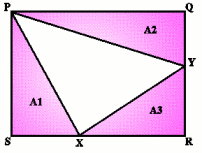#### You may also like### Darts and Kites

Explore the geometry of these dart and kite shapes!### Pent

The diagram shows a regular pentagon with sides of unit length. Find all the angles in the diagram. Prove that the quadrilateral shown in red is a rhombus.### Pentakite

Given a regular pentagon, can you find the distance between two non-adjacent vertices?

# Golden Thoughts

##### Age 14 to 16Challenge Level
Consider the following diagram:Given that the areas of $A1$, $A2$ and $A3$ in the above diagram are equal, show that $${RX\over XS} = {RY\over YQ} = {{\sqrt{5}+ 1}\over 2}$$ so that the points $X$ and $Y$ divide the sides of the rectangle in the golden ratio.